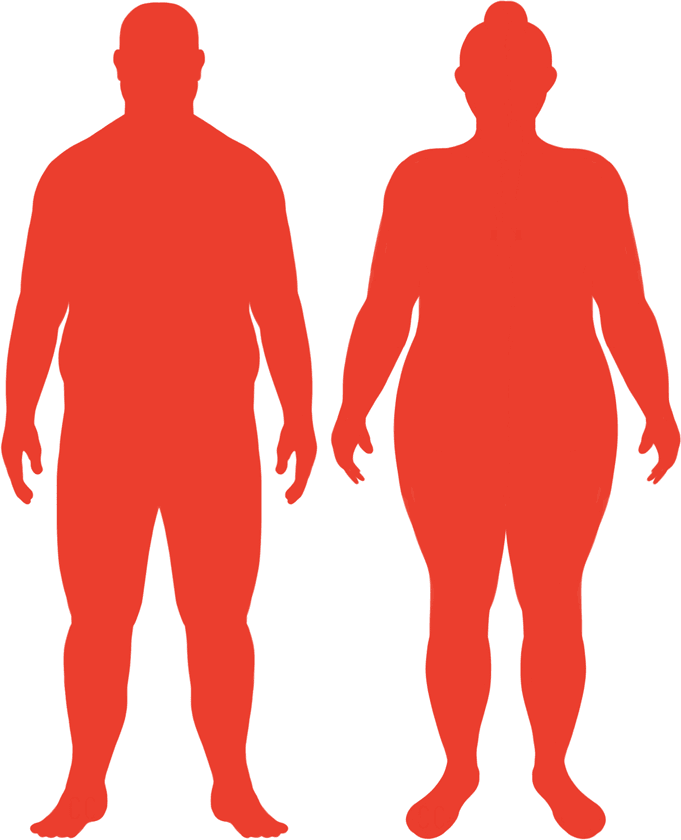# BMI Calculator

Result for 5'11" and 255 lbs.
Change Here.35.56

### Obese BMI

At 5'11" and 255 Pounds your BMI is 35.56

### BMI Scale

 Under Normal Over Obese
-40 lbs
to reach an
overweight bmi classification.
##### Overweight BMI Ends:
215.1 lbs
-76 lbs
to reach a
normal bmi classification.
179.2 lbs

### BMI Grading Table2 for Height 5'11"

 Weight Weight Class Below 132.6 lbs Underweight 132.6 lbs - 179.2 lbs Normal Weight 179.2 lbs - 215.1 lbs Overweight 215.1 lbs and Above Obese

### Weight Loss

If you were seeking a normal BMI weight of 179.2lbs, and you lost two pounds a week4, then you could reach a normal BMI in
38 Weeks
Or to be considered overweight, a goal weight of 215.1 lbs could be reached in
20 Weeks

### Obese BMI Silhouette### Ideal Weight for a 5 foot 11 Male or Female2

The ideal weight range using the BMI for a male or female with a height of 5'11" is

### Between

132.6 lbs

and
179.2 lbs
How to Calculate BMI3 for 5'11" and 255 lbs
(5 x 12) + 11 = 71
(255 / (71 x 71)) x 703
= 35.561396548304
5 = foot | 11 = inch | 255 = weight lbs

### Conversion

• 5 foot 11 = 71 inches
• 5 foot 11 = 180.34cm
• 255 pounds = 18 stone 3 pounds
• 255 pounds = 115.67 kg
• 255 pounds = 115666.05 grams

### 5'11" and 255 lbs Summary

What is the BMI for a 5'11" and 255 lbs female? 35.56 BMI, Obese.
What is the BMI for a 5'11" and 255 lbs male? 35.56 BMI, Obese.
What is the ideal weight for a 5'11" female? Between: 132.6lbs and 179.2lbs
What is the ideal weight for a 5'11" male? Between: 132.6lbs and 179.2lbs
If I am 5ft 11in and weigh 255 lbs, is that a good weight for my height? Under the BMI classification, 255 lbs is classed as being Obese.